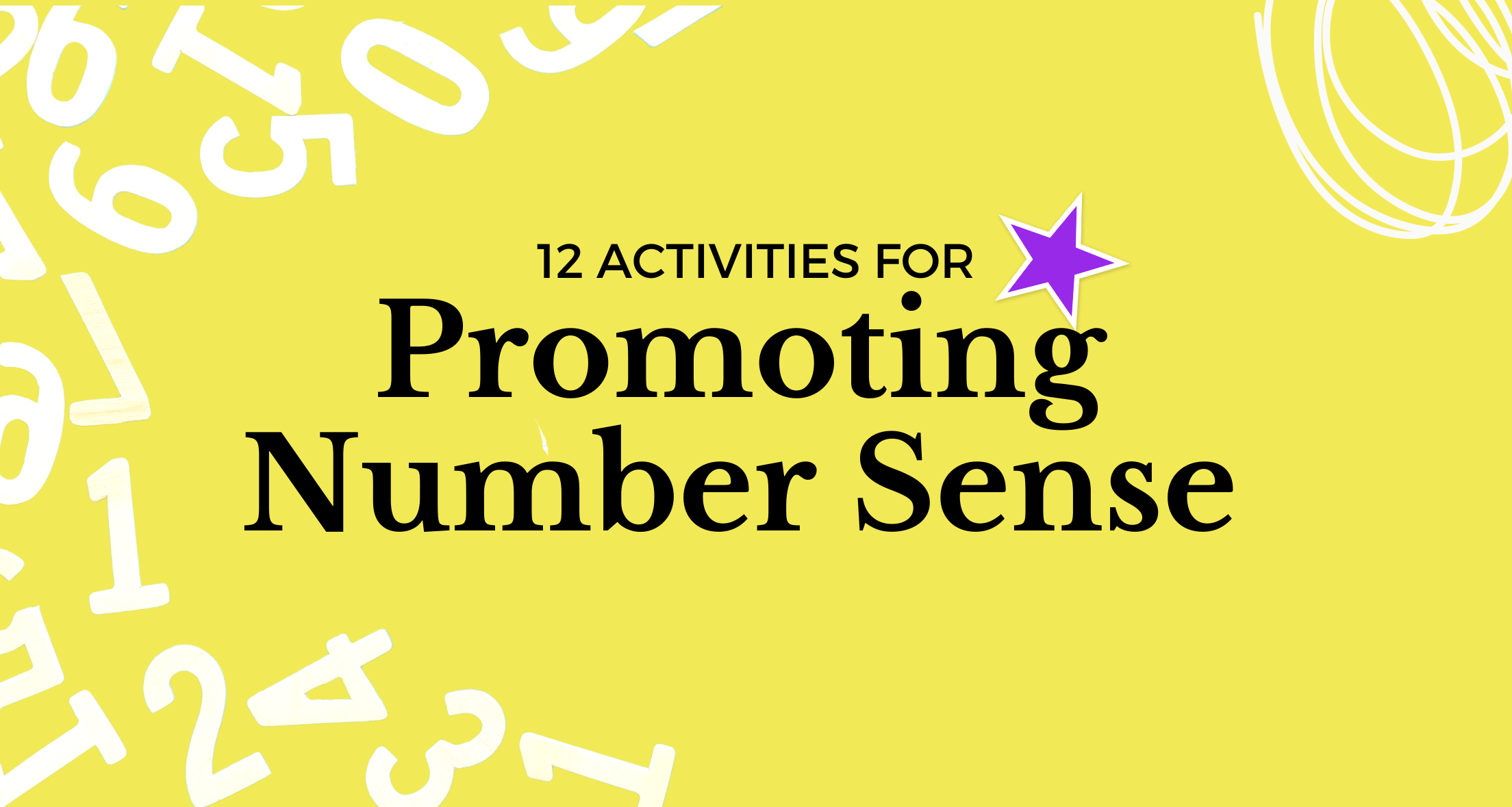### Building number sense lays the foundation for flexible and fluent thinking in all aspects of math. It is a group of essential skills that enable children to use and understand numbers, including:

• Abstract Counting
• Counting objects
• Comparing objects (more, less, equal)
• Reading and writing numbers (7 and seven)
• Matching numbers to a set of objects
• Identifying numbers that come before, after, or in between other numbers
• Ordering numbers (greatest to least and least to greatest)
• Identifying the relationship between ”parts to whole” and “whole to parts”
• Operations with numbers: adding, subtracting, multiplying and dividing
• Estimation

Number sense is not something that can be taught outright. It is something that is gradually fostered and developed when children connect numbers to real-life situations. The more children use and experience numbers in the real world to make connections, the more fluent and flexible they become with numbers. Here are some ideas to promote number sense at home:

### 1. Count

Have your child count anything and everything!

• How many steps do you take from the door to the car?
• How many grapes are on your plate?

### 2. Counting on

• Have your child add two groups of objects together. For example, if there are 4 objects in one group and 3 in the other, then they should put the four in their hand and say “four” then count on “five, six, seven”

### 3. Make 10

• Can you make a group of 10 beans?
• How else can you make 10?
• What if there are 3 more in this group?
• How many more/less objects do I need to make 10?

• Count by 2s
• Count by 5s
• Count by 10s

### 5. Make a number using objects

• How many ways can you make 5 using two groups?
• 1+4, 2+3, 0+5, 4+1, 3+2, 5+0
• How many ways can you make 27 using two groups?

### 6. Group items by characteristics and count/compare

• How many green M&Ms are in the bag?
• Are there more red or blue M&Ms?
• How many green and red M&Ms are there together?

### 7. Estimate

Have your child guess how many objects there are. Then count how many are actually there. Were there more or less than your guess?

• How many chips do you think are in your bag?
• How long do you think it will take in the drive-thru?
• How many steps do you think are from the living room to the bathroom?

### 8. Even or odd?

Have your child sort objects into pairs.

• How many pairs can you make?
• Are there any left over without a pair? These are odd numbers!!

### 9. What number am I?

• I am more than 5 but less than 10.
• I am an odd number.
• If you take away 2 there will be 5 left.

### 10. Make change

Have your child count the change at the store.

### 11. Cook together

• Measure ingredients in measuring cups and spoons.
• Ask which is larger ½ or ⅓?
• How many ½ cups are in a cup?
• How many ¼ cups are in a ½ cup?
• If I have 3 cookies, how many are left?